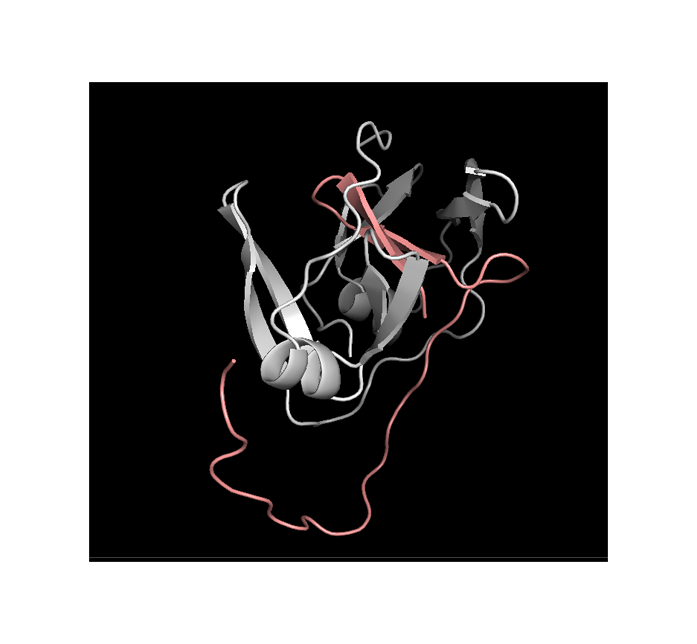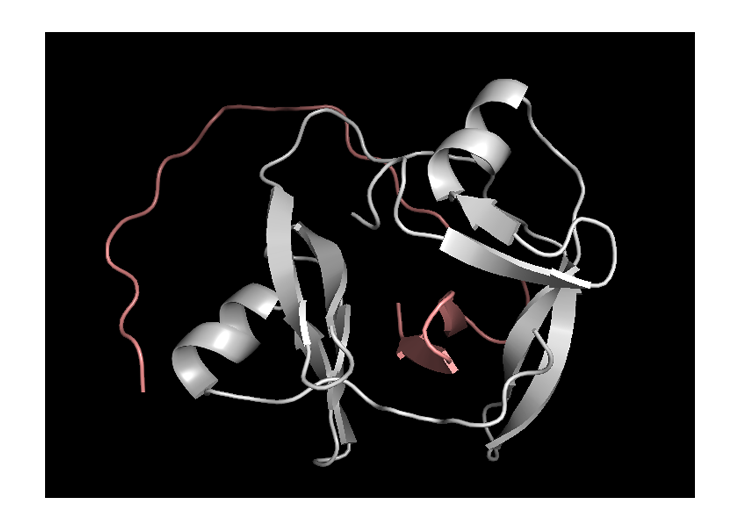# Team:Moscow City/Model

<!DOCTYPE html>

Modelling
Report on b-Lactamase and intein fragments halves protein-protein interaction modeling
1.. Template modeling
Sequence:
SGAGERGSRGIIAALGPDGKPSRIVVIYTTGSQATMDER
NRQIAEIGASLIKHWHPETLVKVKDAEDQLGARVGYIELD
LNSGKILESFRPEERFPMMSTFKVLLCGAVLSRIDAGQEQ
LGRRIHYSQNDLVEYSPVTEKHLTDGMTVRELCSAAITM
SDNTAANLLLTTIGGPKELTAFLHNMGDHVTRLDRWEPE
LNEAIPNDERDTTMPVAMATTLRKLLTG
The molecule is “cut” between: Trp93 and His94
Template modeling performed by Swiss-model:
This structure can be trusted as sequence identity with the template is extremely high – 98.71%. It is usually considered that template modelling can be to a certain extent accurate when this number is higher than 40%.
The only flaw of the structure is the absence of the first 31 amino acids in it. However, the impact of those amino acids in the studied here halves interaction is negligible.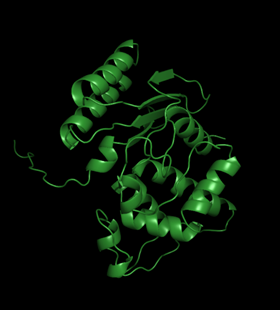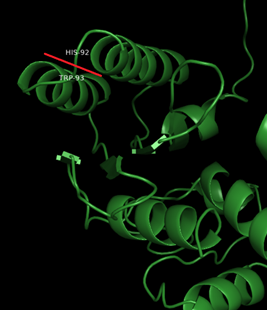2.. Protein-protein docking
Current work is based on the assumption that the beta-Lactamase divided in halves will unite in the same position as in the native protein. To ground this assumption, we have to prove that this position corresponds to the minimum of energy. For this purpose, protein-protein docking was performed. Out of all possible poses (note that approximately 10^9 were processed), the most energetically advantageous turned out to be the two demonstrated below:Green corresponds to the original structure, orange and blue are best docking poses. Even visually it is clear that the structures are similar. However, it is necessary to characterize this similarity quantitatively. RMSD =root mean square deviation serves as such a characteristic. For these structures RMSDs are 0,715 and 0,636 A. These numbers are not negligible, nonetheless small.
At this stage we can assume that native position of the halves is favorable, thus it will lead to beta-Lactamase fermentative activity retrieval and the test working correctly.
REMARK: the bonds between the halves are obviously non-covalent
3.. Molecular dynamics
Molecular dynamics is a method of computer simulation, in most common versions based on solving Newtonian equations of motion for a system of interacting particles. It is important to emphasize that in this method molecules are described mechanically and not as quantum systems. On the scale of biomolecules quantum description makes computations too costly. However, quantum effects are taken into account in a form of ad hoc functional approximations.
The system is described through an empirical potential, or so-called force field. The force field is the system’s potential energy, consisting of chemical bonds, bond angles and bond dihedrals, and non-bonded interactions, such as van der Waals and electrostatic. It is a function of many variables. For the most common task – equilibration of the system – its minimum is sought, as the most stable condition corresponds to the minimum of energy.
In this particular work, molecular dynamics was employed to estimate the binding free energy of the split beta-Lactamase and prove that the process of its reunification is energetically profitable.
The process could be described as:Thus, the most obvious way to calculate binding free energy would be from the process below:However in such a system solvatation energy is by order of magnitude higher, than the binding energy. For this reason, the calculations would take an inordinate amount of time to converge. This is why the calculation is divided according to the following thermodynamic cycle:In this particular scheme molecules are called “receptor” and “ligand”, but it is clear that it is general enough to be employed for two protein molecules which are technically not a receptor and a ligand.
The binding free energy can be calculated as follows: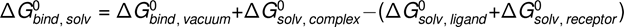A method frequently used to achieve that and utilized in the current work is called MM-PBSA or molecular mechanics Poisson-Bolzman surface area.

In this method, solvation free energies are calculated by solving either the linearised Poisson Boltzman or Generalized Born equation for each of the three states. This gives the electrostatic contribution to the solvation free energy. Hydrophobic interactions are taken into account in an empirical term: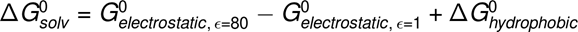By calculating the average interaction energy between receptor and ligand G_vacuum can be obtained. Entropy change upon binding is taken into account if necessary:
In practice, entropy change is usually neglected, except for comparison of states of similar entropy is desired.

To obtain average interaction energies, calculations are performed on a series of uncorrelated snapshots of an equilibrated molecular dynamics simulation.

Molecular dynamics was performed in Amber18 software.

The beta-Lactamase model built in (1) was solvated in a box with periodiс boundary conditions (tip3p water model). As the molecule is fully protein, a protein force field ff14SB was employed. All simulations were run with shake on hydrogen atoms, a 2 fs time step and Langevin dynamics for temperature control. The system was minimized and heated for 50 ps. Then, density equilibration for 50 ps with weak restraints on the complex was performed. It was followed by a 2500 ps constant pressure equilibration at 300K.

Then, the production simulation was run for 20000 ps (20 ns) with snapshots taken every 10 ps. All the simulations in total took approximately 80 hours on Intel® Core™ i5-4570 CPU @ 3.20GHz × 4 and a graphic unit NVIDIA GeForce GTX 1060 3GB/PCIe/SSE2. The 2000 snapshots were then analysed by MMPBSA.py. Here are the results of Poisson Bolzman calculations:From the negative total binding free energy -20.84±0.38 kcal/mol it is clearly seen that such a protein-protein complex in water solution is favorable. However, the result does not equal the real binding free energy since entropy contribution to the binding was not estimated.
4. N-intein C-intein report
(DNA-polymerase subunit alfa)
4.1 C-intein N-intein tertiary structure prediction by AlphaFold2
First figure demonstrates how many similar structures were found and their coverage. It is clear that wider coverage means nore identity with the given structure, and thus more structures with known folding will be found. This correlation is very well seen for C-int: 8000 vs 2500.

lDDT - local Distance Difference Test

The test measures how well local interactions from the template model are kept in tha assesed one. In the calculation, distances between all pairs of atoms in the template structure lying closer than certain radius (called inclusion radius) and not belonging to the same residue, are taken into account. The distance in the assesed model is considered to be preserved if it is the same as the template's inside the inclusion radius.

lDDT higher than 80 is a good score, for this reason the structure can be trusted.

Taking into account all these consuderations, models with the lowest alignment score and highest lDDT were chosen for further analysis.
N-int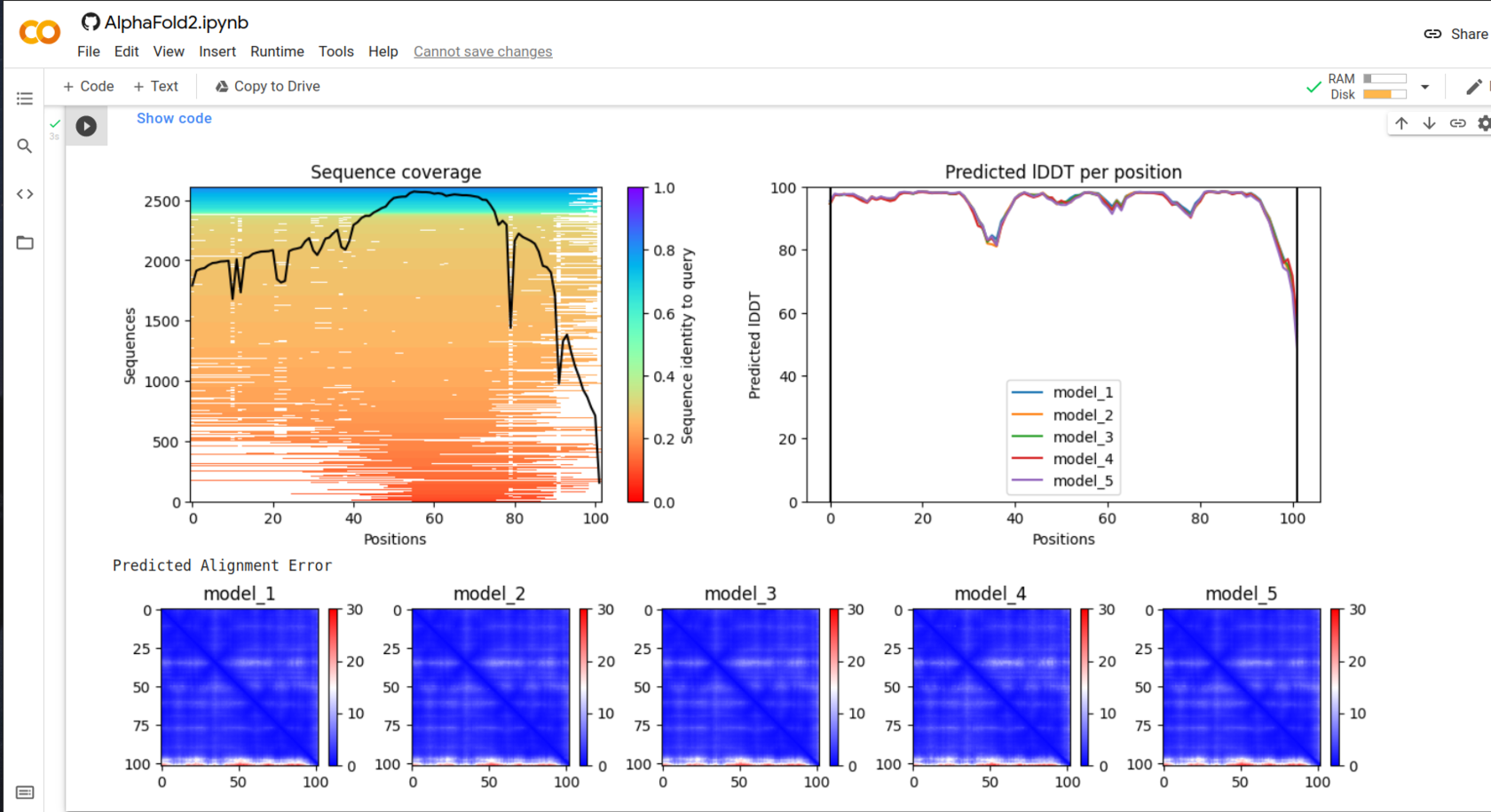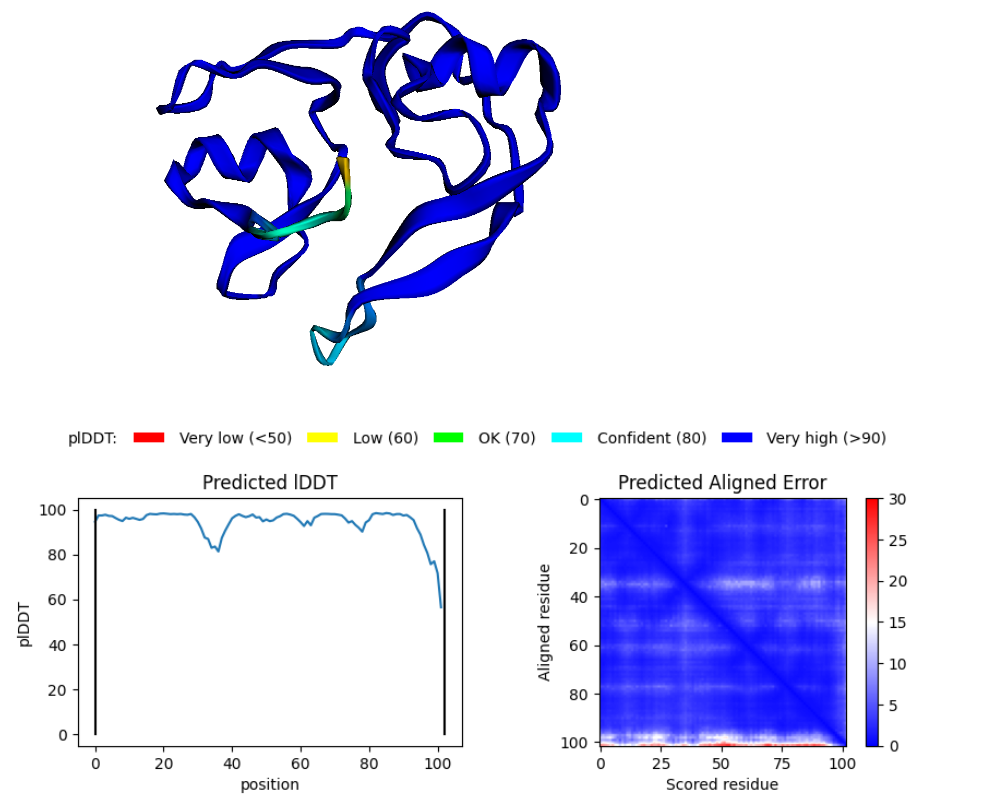Blue parts of the structure indicate segments that can be higly trusted.
C-int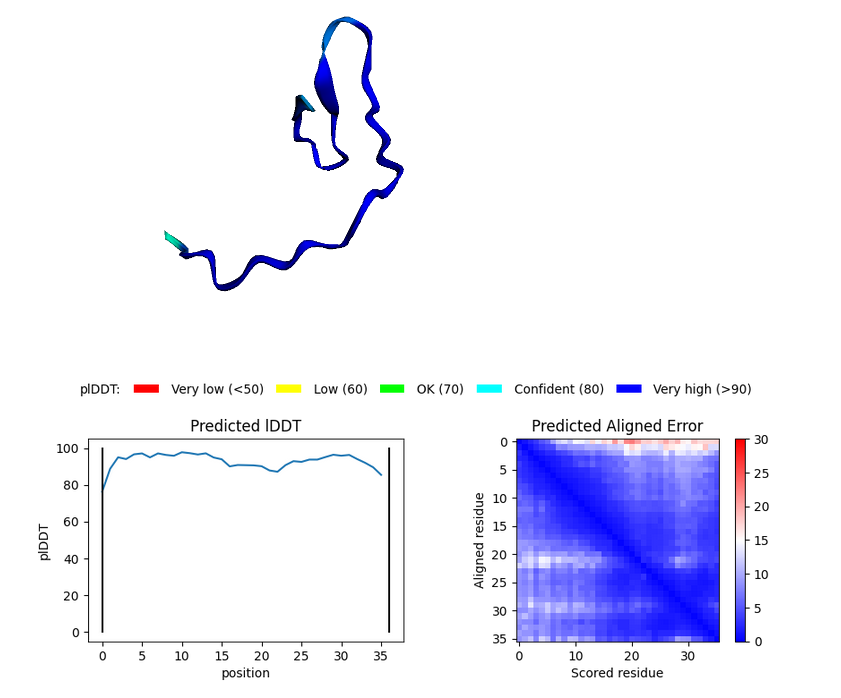4.2 ClusPro 2.0 docking
Complex number 0 has the lowest energy thus is the most stable. This complex can be used for further investigation.The most probable protein disposition: